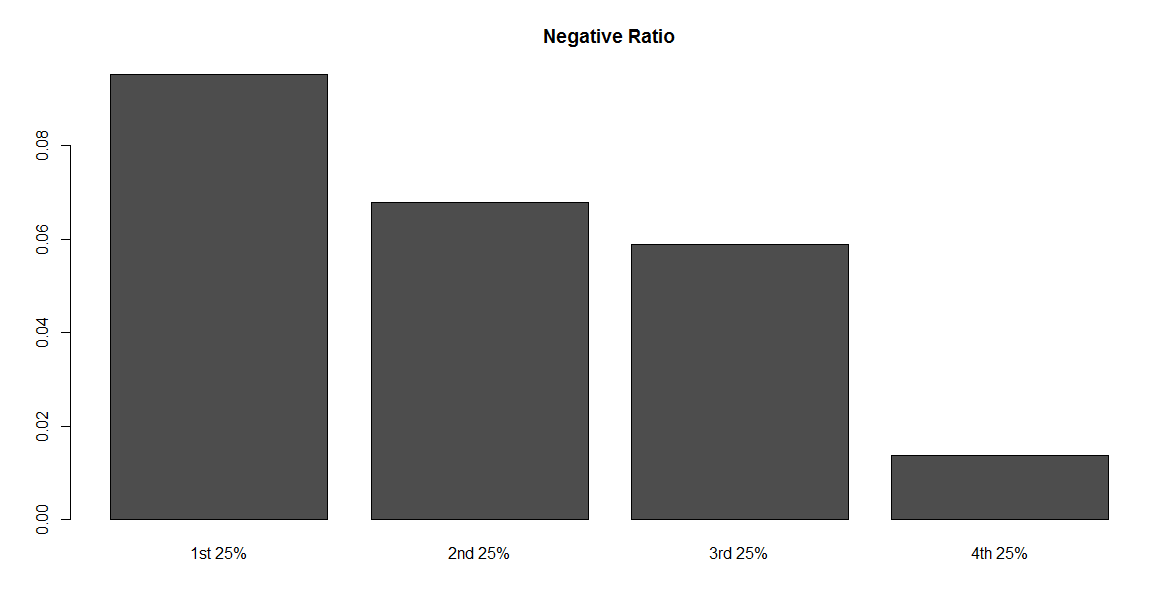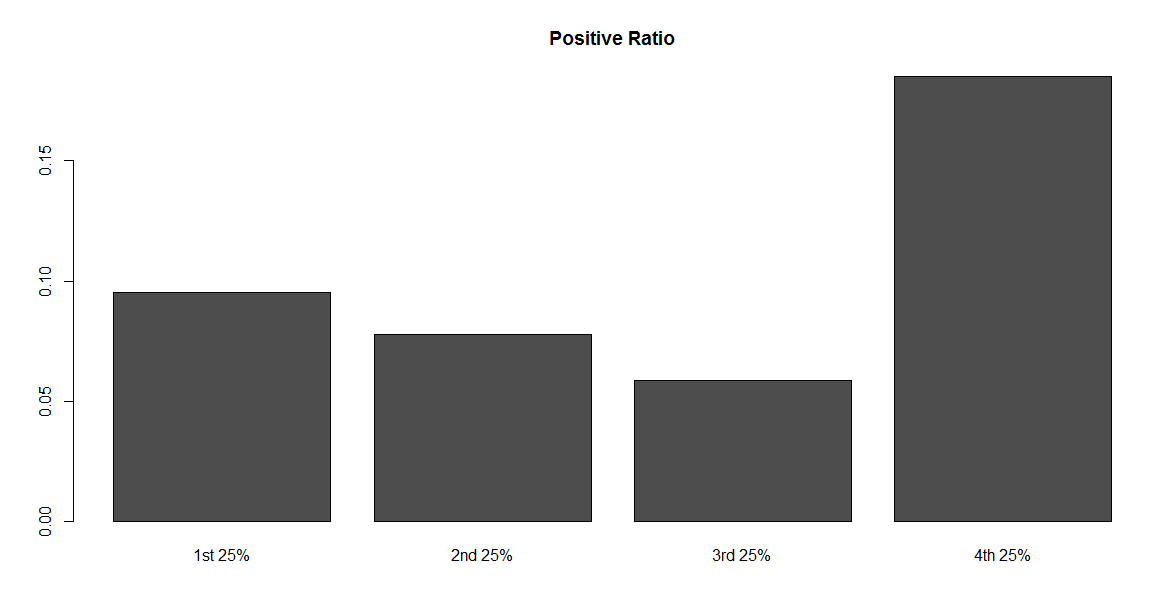# Text Mining (Sentiment Analysis) Lab homework

—————— Chapter 15: Happy Words? ——————

pos <- “positive-words.txt”

neg <- “negative-words.txt”

p <- scan(pos, character(0),sep = “n”)

n <- scan(neg, character(0),sep = “n”)

p <- p[-1:-29]

n <- n[-1:-30]

totalWords <- sum(wordCounts)

words <- names(wordCounts)

matched <- match(words, p, nomatch = 0)

matched

p

words

mCounts <- wordCounts[which(matched != 0)]

length(mCounts)

mWords <- names(mCounts)

nPos <- sum(mCounts)

nPos

matched <- match(words, n, nomatch = 0)

nCounts <- wordCounts[which(matched != 0)]

nNeg <- sum(nCounts)

nWords <- names(nCounts)

nNeg

length(nCounts)

totalWords <- length(words)

ratioPos <- nPos/totalWords

ratioPos

ratioNeg <- nNeg/totalWords

# Instructions

Conduct sentiment analysis on MLK’s speech to determine how positive/negative his speech was. Split his speech into four quartiles to see how that sentiment changes over time.Create two bar charts to display your results.

``# Add your library below.``

# Step 1 – Read in the positive and negative word files

## Step 1.1 – Find the files

Find two files (one for positive words and one for negative words) from the UIC website. These files are about halfway down the page, listed as “A list of English positive and negative opinion words or sentiment words”. Use the link below:

Save these files in your “data” folder.

``# No code necessary; Save the files in your project's data folder.``

## Step 1.2 – Create vectors

Create two vectors of words, one for the positive words and one for the negative words.

``# Write your code below.``

## Step 1.3 – Clean the files

Note that when reading in the word files, there might be lines at the start and/or the end that will need to be removed (i.e. you should clean your dataset).

``# Write your code below.``

# Step 2: Process in the MLK speech

## Step 2.1 – Find and read in the file.

Find MLK’s speech on the AnalyticTech website. Use the link below:

Read in the file using the XML package. Otherwise, cut and paste the document into a .txt file.

``# Write your code below.``

## Step 2.2 – Parse the files

If you parse the html file using the XML package, the following code might help:

``````# Read and parse HTML file

doc.html = htmlTreeParse('http://www.analytictech.com/mb021/mlk.htm',
useInternal = TRUE)

# Extract all the paragraphs (HTML tag is p, starting at
# the root of the document). Unlist flattens the list to
# create a character vector.

doc.text = unlist(xpathApply(doc.html, '//p', xmlValue))

# Replace all n by spaces
doc.text = gsub('\n', ' ', doc.text)

# Replace all r by spaces
doc.text = gsub('\r', ' ', doc.text)``````
``# Write your code below, if necessary.``

## Step 2.3 – Create a term matrix

Create a term matrix.

``# Write your code below.``

## Step 2.4 – Create a list

Create a list of counts for each word.

``# Write your code below.``

# Step 3: Positive words

Determine how many positive words were in the speech. Scale the number based on the total number of words in the speech. Hint: One way to do this is to use `match()` and then `which()`.

``# Write your code below.``

# Step 4: Negative words

Determine how many negative words were in the speech. Scale the number based on the total number of words in the speech.
Hint: This is basically the same as Step 3.

``# Write your code below.``

# Step 5: Get Quartile values

Redo the “positive” and “negative” calculations for each 25% of the speech by following the steps below.

## 5.1 Compare the results in a graph

Compare the results (e.g., a simple bar chart of the 4 numbers).
For each quarter of the text, you calculate the positive and negative ratio, as was done in Step 4 and Step 5.
The only extra work is to split the text to four equal parts, then visualize the positive and negative ratios by plotting.

The final graphs should look like below:HINT: The code below shows how to start the first 25% of the speech. Finish the analysis and use the same approach for the rest of the speech.

``````# Step 5: Redo the positive and negative calculations for each 25% of the speech
# define a cutpoint to split the document into 4 parts; round the number to get an interger
cutpoint <- round(length(words.corpus)/4)

# first 25%
# create word corpus for the first quarter using cutpoints
words.corpus1 <- words.corpus[1:cutpoint]
# create term document matrix for the first quarter
tdm1 <- TermDocumentMatrix(words.corpus1)
# convert tdm1 into a matrix called "m1"
m1 <- as.matrix(tdm1)
# create a list of word counts for the first quarter and sort the list
wordCounts1 <- rowSums(m1)
wordCounts1 <- sort(wordCounts1, decreasing=TRUE)
# calculate total words of the first 25%``````
``# Write your code below.``

# 5.2 Analysis

What do you see from the positive/negative ratio in the graph? State what you learned from the MLK speech using the sentiment analysis results:

[ Type your analysis here. ]

## Calculate the price of your order

550 words
We'll send you the first draft for approval by September 11, 2018 at 10:52 AM
Total price:
\$26
The price is based on these factors:
Number of pages
Urgency
Basic features
• Free title page and bibliography
• Unlimited revisions
• Plagiarism-free guarantee
• Money-back guarantee
On-demand options
• Writer’s samples
• Part-by-part delivery
• Overnight delivery
• Copies of used sources
Paper format
• 275 words per page
• 12 pt Arial/Times New Roman
• Double line spacing
• Any citation style (APA, MLA, Chicago/Turabian, Harvard)

# Our guarantees

Delivering a high-quality product at a reasonable price is not enough anymore.
That’s why we have developed 5 beneficial guarantees that will make your experience with our service enjoyable, easy, and safe.

### Money-back guarantee

You have to be 100% sure of the quality of your product to give a money-back guarantee. This describes us perfectly. Make sure that this guarantee is totally transparent.

### Zero-plagiarism guarantee

Each paper is composed from scratch, according to your instructions. It is then checked by our plagiarism-detection software. There is no gap where plagiarism could squeeze in.

### Free-revision policy

Thanks to our free revisions, there is no way for you to be unsatisfied. We will work on your paper until you are completely happy with the result.